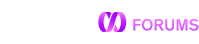# Current week and last 4 weeks

I am trying to calculate the current week and last 4 weeks for a query and this is one of the logic i used previously with SQL but i donot see it working with MEMSQL .

In SQL i used getdate and changed the function here . Any ideas what might be the issue here
select

``````DATE,

``````

from

``````(

select	DATE = now()	union all

select	DATE = now()-7	union all

select	DATE = now()-(7*2)	union all

select	DATE = now()-(7*3)	union all

select	DATE = now()-(7*4)

) table1
``````

order by table1.DATE desc

To do what you want you have to specify a unit of time e.g. `now() - interval 7 days`.

I did make that change
select DATE,

from

``````(

select	DATE = now()	union all

select	DATE = now()- (INTERVAL 1 week)	union all

select	DATE = now()-(INTERVAL 2 week) union all

select	DATE = now()-(INTERVAL 3 week)	union all

select	DATE = now()-(INTERVAL 1 week)

) table1
``````

order by

``````table1.DATE desc
``````

It tells me the error is at date_add(dd,(date_sub(dd,‘17530107’,table1.DATE)/7)*7,‘17530107’)

Your syntax `WeekStartDate = date_add(...)` and `DATE = now()` is not valid in MemSQL. (What system are you coming from that has that syntax?)

Try `date_add(...) as WeekStartDate` and `now() as DATE`.

Also since you said the goal was to calculate the current week, have you tried https://docs.memsql.com/v6.8/reference/sql-reference/date-and-time-functions/week/?